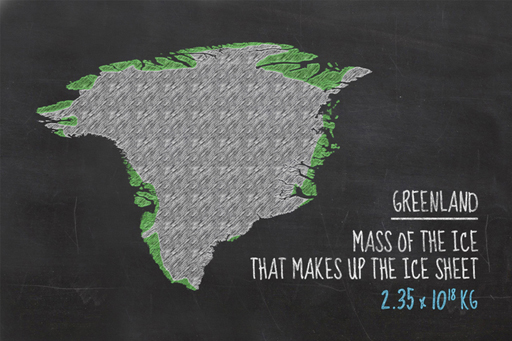Basic science: understanding numbers

Start this free course now. Just create an account and sign in. Enrol and complete the course for a free statement of participation or digital badge if available.

Why the Greenland ice sheet matters to usFigure 9 What would happen if this much ice were to melt?

In the previous sections, you’ve seen how areas, volumes and density are calculated, using the Greenland ice sheet as an example. You saw that the mass of the ice that makes up the ice sheet is about 2.35 × 1018 kg.

If the Greenland ice sheet were to completely melt, the water would flow into the oceans and add to them. If enough water is added, sea levels will rise noticeably. How much they will rise is something you can now work out using what you have learned so far.

The oceans cover about 70.9% of the Earth and the Earth has an area of about 510,082,000 km2. You will learn more about percentages later in the course, so for now we’ll tell you that 70.9% of 510,082,000 is 361,650,000 km2. (We’ve rounded this number – you’ll also learn about rounding later in the course.)

So, you have a figure for both the area of the oceans and the mass of ice. You now need to convert that mass of ice into a volume of water.

Water and ice have different densities; remember that ice is less dense? But the mass remains the same, so the mass of the water is the same as the mass of the ice.

Water has a density of 1000 kg/m3. The mass of water is 2.35 x 1018 kg, so that gives a volume of 2.35 × 1015 m3 or 2,352,105,000,000,000 cubic metres. You could work it all out in cubic metres but cubic kilometres makes more sense for such a large volume. Remember that there are 1,000,000,000 (109) cubic metres in a cubic kilometre, so if you convert the volume of water to cubic kilometres it becomes a billion times smaller, or 2,352,105 km3.

You now have all you need to work out an estimate for the rise in sea level from the melting of the Greenland ice sheet. The change in sea level is just the volume of water added to the oceans divided by the surface area of those oceans.

2,352,105 km3 divided by 361,650,000 km2 is 0.0065 km, or 6.5 metres. The official estimate is influenced by other factors – for example, the oceans get bigger when sea levels rise, which we haven’t accounted for – but 6.5 metres will be pretty close – try searching the internet for the official estimate.

Is the official estimate more or less than you were expecting? How would this affect coastal areas you have visited?